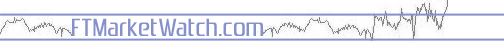# Investment Dictionary, In Category [Technical Analysis]

Alpha Analysis Arithmetic mean Arms Index Ascending bottoms Ascending tops Autocorrelation Autoregressive Base Beta Blow-off top Bollinger bands Bottom Breadth-of-market theory Candlestick charts Chart Charting Chartist Coefficient of determination Congestion Correlation Correlation coefficient Cost of sales Covariance Cup and handle Descending bottoms Descending tops Detrend Double bottom Double top Double top breakout Down volume Elliott Wave Theory Epsilon Flag Forecast Fourier analysis Head and shoulders High-low index Inflection point Intermediate-term Interpolation Inverse relationship Linear chart Linear regression Logarithmic chart Lognormal distribution MACD Market analysis McClellan Oscillator Mean Mean return Median Medium-term Milner analysis Mode Monte Carlo Moving Average Convergence/Divergence Multiple NOI Normal distribution Odd-lot theory On-balance volume Oscillator Overbought Overbought/oversold indicator Oversold Pattern Pennant Plateau Point-and-figure chart Probability distribution Profit ratio Projection Ratio Ratio analysis Regression analysis Regression equation Relative Strength Index Resistance Return on Capital Employed Rising bottoms Risk ROC ROCE ROTA RSI Saucer Serial correlation Signal Simple moving average Simulation Standard deviation Stochastic Support Technical analysis Technical analyst Technical indicator Technicals Technician Test Top Top out Trading Index Trendline Triangle TRIN Triple bottom Up volume Variance Vertical line charting Volatility Wedge W formation